# CLASS 10 MATH NCERT SOLUTIONS FOR CHAPTER – 1 REAL NUMBERS EX – 1.4

## Real Numbers

Question 1.
Without actually performing the long division, state whether the following rational numbers will have a terminating decimal expansion or non-terminating repeating decimal expansion: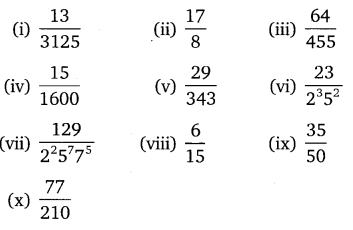Solution: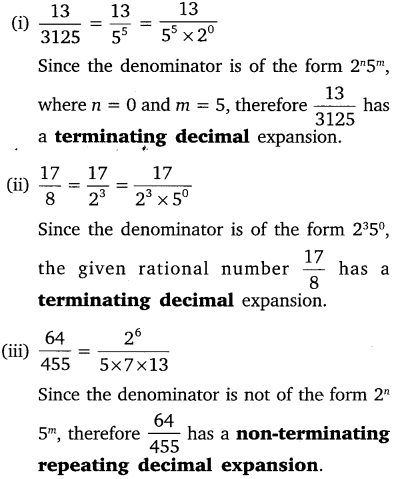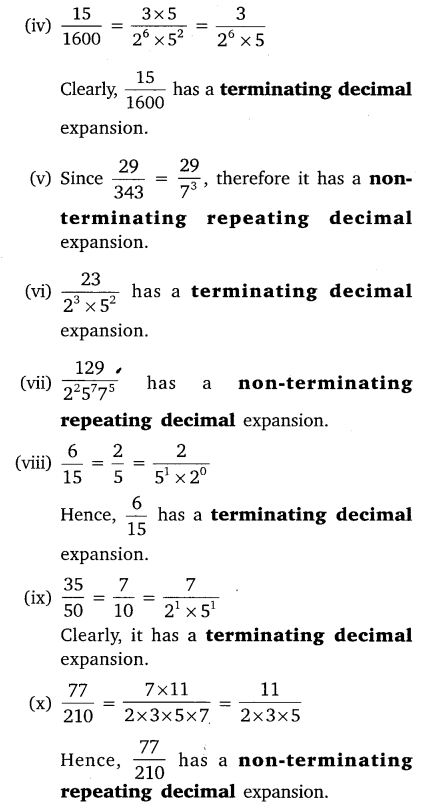Question 2.
Write down the decimal expansions of those rational numbers in the question 1, which have terminating decimal expansions.

Solution: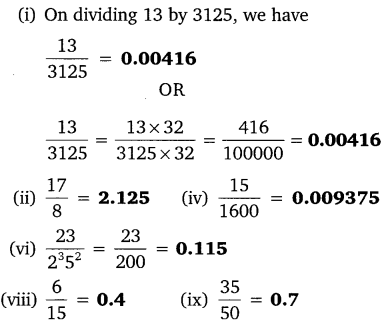Question 3.
The following real numbers have decimal expansions as given below. In each case, decide whether they are rational or not. If they are rational and of the form$\frac { p }{ q }$, what can you say about the prime factors of q ?

(i) 43. 123456789
(ii) 0.120120012000120000…
(iii) 43.$\overline { 123456789 }$

Solution:
(i)
43.123456789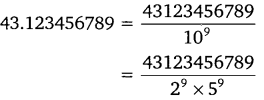Here, the denominator is of the form 2m5n.
Hence, the number is a rational number, specifically a terminating decimal.

(ii) Since the given decimal number is non ­terminating non-repeating, it is not rational

(iii) Since the given decimal number is non terminating repeating, it is rational, but the denominator is not of the form 2m5n.

#### CLASS 10 MATH NCERT SOLUTIONS FOR CHAPTER – 2 Polynomials Ex – 2.1

Lorem ipsum dolor sit amet, consectetur adipiscing elit. Phasellus cursus rutrum est nec suscipit. Ut et ultrices nisi. Vivamus id nisl ligula. Nulla sed iaculis ipsum.

Company Name